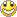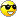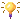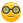Some new stuff
1. Arduino Park Control
2. Arduino USB2serial programming the bare bone
3. Arduino Projects Videos added
4. Arduino Xbee projects
5. Arduino dB Meter
6. Trying to get the IOIO going.
7. IOIO is going....bluetooth8. Attiny 2313 bluetooth bee RC car
9. Compass and temperature added
10. AVR programmer
11. USB2Serial and Ethernet POE
12. Arduino LPG Sensor
13. Program the Arduino Mini 05
14. Lipo Rider Pro in action
15. EZ Robot Builder kit arrived 10 Nov 2012
16. Arduino VA Meter!
17. 05 Nov 2012 Project Sentry Gun.
18. Attiny85 PIR Sensor 10 Dec 2012
19. 0-30VDC3A and 2-28VDC10A bench PSU's20. 19 Dec 2012 Arduino Voltmeter LCD
21. 29 Dec 2012 Arduino Tachometer&Speedometer LCD
22. 03 Jan 2013 Arduino AC Phase Control.
23. 18 Jan 2013 Arduino Distance Meter
24. 22 Jan 2013 Arduino Digital Clock and Date
25. 31 Jan 2013 Arduino Digital Clock and Date 8x2 LCD
26. Feb 2013 LCD and Backpack
27. Feb 2013 7 Segment Serial Clock Sparkfun. Distance Sensor with "newping" library
28. Feb 2013 Lelo Remote
29. 01/03/2013 Arduino Adafruit 7 Seg Digital Clock with RTC and backpack
30. Arduino Frequency Meter 26/03/2013
31. Another RC  Car controlled by Arduino 06/04/2013
32. My quadcopter project update 25 April 2013
33. My Aqua Quad Copter Flying 26 April 201334. Another monster RC truck;strong one! 11July201335. X-Frame Quad Copter 31 July 201336. Arduino GSM Sheild added.28/8/2013
37. 1.8inch TFT screen 13/09/201338. 04/11/2014 added PID Soldering Iron Control
39. Added bluetooth servo control 04/11/2014
40. ESP8266 first try.16/03/2015
41. Arduino Uno Quadcopter 19 May 2015
42. IOT WemosD1 Amp MeterArduino Capacitance Meter 15 Jan 2013.

I altered the codes both LCD and Serial Monitor available on the net to work with LCD Keypad and Arduino.

/*  RCTiming_capacitance_meter

*   Paul Badger 2008

*  Demonstrates use of RC time constants to measure the value of a capacitor

*

* Theory   A capacitor will charge, through a resistor, in one time constant, defined as T seconds where

*    TC = R * C

*

*    TC = time constant period in seconds

*    R = resistance in ohms

*    C = capacitance in farads (1 microfarad (ufd) = .0000001 farad = 10^-6 farads )

*

*    The capacitor's voltage at one time constant is defined as 63.2% of the charging voltage.

*

*  Hardware setup:

*  Test Capacitor between common point and ground (positive side of an electrolytic capacitor  to common)

*  Test Resistor between chargePin and common point

*  220 ohm resistor between dischargePin and common point

*  Wire between common point and analogPin (A/D input)

*/

#include <LiquidCrystal.h>

LiquidCrystal lcd(8, 9, 4, 5, 6, 7);

#define analogPin      1          // analog pin for measuring capacitor voltage

#define chargePin      3         // pin to charge the capacitor - connected to one end of the charging resistor

#define dischargePin   2         // pin to discharge the capacitor

#define resistorValue  10000.0F   // change this to whatever resistor value you are using

// F formatter tells compliler it's a floating point value

unsigned long startTime;

unsigned long elapsedTime;

float microFarads;                // floating point variable to preserve precision, make calculations

void setup(){

lcd.begin(16, 2);

pinMode(chargePin, OUTPUT);     // set chargePin to output

digitalWrite(chargePin, LOW);

lcd.clear();

lcd.setCursor(0, 0);

lcd.print("Cap Meter");

lcd.setCursor(0,1);

lcd.print("Range:1nF-999uF");

delay(2000);

pinMode(chargePin, OUTPUT);     // set chargePin to output

digitalWrite(chargePin, LOW);

Serial.begin(9600);             // initialize serial transmission for debugging

}

void loop(){

digitalWrite(chargePin, HIGH);  // set chargePin HIGH and capacitor charging

startTime = millis();

while(analogRead(analogPin) < 648){       // 647 is 63.2% of 1023, which corresponds to full-scale voltage

}

elapsedTime= millis() - startTime;

// convert milliseconds to seconds ( 10^-3 ) and Farads to microFarads ( 10^6 ),  net 10^3 (1000)

microFarads = ((float)elapsedTime / resistorValue) * 1000;

Serial.print(elapsedTime);       // print the value to serial port

lcd.clear();

lcd.setCursor(0,0);

lcd.print(elapsedTime);

Serial.print(" mS    ");         // print units and carriage return

lcd.setCursor(6,0);

lcd.print("mS");

delay(500);

if (microFarads > 1){

Serial.print((long)microFarads);       // print the value to serial port

lcd.setCursor(0,1);

lcd.setCursor(6,1);

Serial.println(" microFarads");         // print units and carriage return

lcd.print("uF");

delay(500);

}

else

{

// if value is smaller than one microFarad, convert to nanoFarads (10^-9 Farad).

// This is  a workaround because Serial.print will not print floats

nanoFarads = microFarads * 1000.0;      // multiply by 1000 to convert to nanoFarads (10^-9 Farads)

Serial.print((long)nanoFarads);         // print the value to serial port

lcd.setCursor(0,1);

Serial.println(" nanoFarads");          // print units and carriage return

lcd.setCursor(6,1);

lcd.print("nF");

delay(500);

}

/* dicharge the capacitor  */

digitalWrite(chargePin, LOW);             // set charge pin to  LOW

pinMode(dischargePin, OUTPUT);            // set discharge pin to output

digitalWrite(dischargePin, LOW);          // set discharge pin LOW

while(analogRead(analogPin) > 0){         // wait until capacitor is completely discharged

}

pinMode(dischargePin, INPUT);            // set discharge pin back to input

}